# How to calculate cumulative resultsHi,everyone.

I would like to calculate the cumulative result of a line item.

I think I should use the TIMESUM function, but I don't understand how to use it.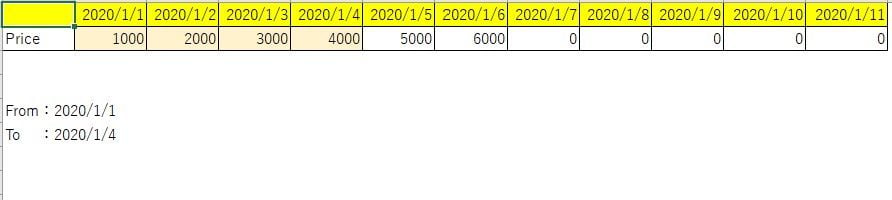I want to get the cumulative result from the date of FROM to the date of TO. Could you tell me the best solution?

Best regards.

Taku

Tagged:

•If you want to achieve same result through the Timesum formula then follow the below step:

1.  Create a system module to select the " From & To Date"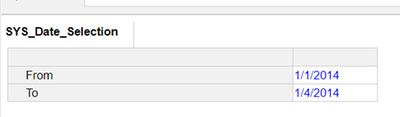2. I have calculated in the same module .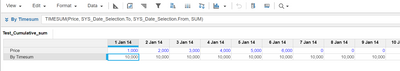better to create another module without time dimension and use timesum formula.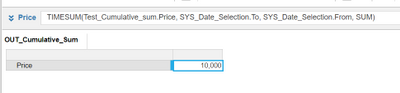Note: TIMESUM is appropriate when the result has no time dimension.

Thanks

Akhtar

•Hi @taku.s,

Here assuming that Time dimension in Module is in Months, also the start period and end period is also in Month format, you could use the Timesum formula as below...

Timesum(Price, From, To )

•Hi, @Rashmih

I tried it and I couldn't. Because "From" And "To" are Date format.

Could you tell me another good solution?

Best regards.

Taku

•Could you please let me know the time dimension in the module..

If the numbers are to be summed by month, then we can convert the From and To date to Month and then use it in the formula.

Period Month = PERIOD(Date)
where the result (Period Month) is time period-formatted as Month and the Date source line item is formatted as Date.

Hope this helps...
•You can try this:

1. Create a system module to select the " From & To Date"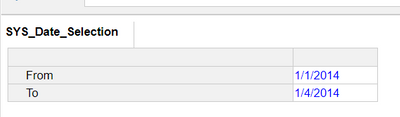2. In your module just add two line item one to check the time range and second to cumulative price: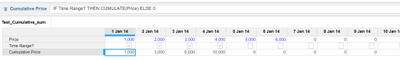now you can write a formula" IF Time Range? THEN CUMULATE(Price) ELSE 0"

Hope this helps!

Thanks

Akhtar

•I would use TIMESUM in this case as you aren't looking to calculate in every period which CUMULATE will do.

Here is a link to the Anapedia article for Timesum, the above is correct you will need to convert your From and To dates to periods using the period function and you will need to do this on separate line items as you can't nest period() within a Timesum function

•Hi,@Akhtar.shahbaz

I was able to get the result I was looking for.

And I understand how to use TIMESUM function.

Thank you so much!!

Best regards.

Taku